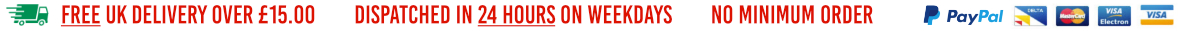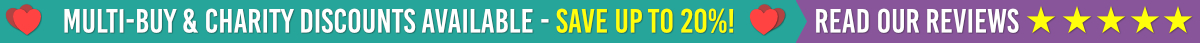Have a question?
FREE UK DELIVERY OVER £15HOT ProductsONLINE SHOPInformation

Black
Brown
Burgundy
Cream
Dark Blue
Forest Green
Green
Grey
Light Blue
Light Pink
Lilac
Orange
Purple
Red
Royal Blue
Teal
Vivid Blue
White
Yellow
Metallic Gold
Metallic Silver
Vibrant/Neon Green
Vibrant/Neon Orange
Vibrant/Neon Pink
Vibrant/Neon Yellow

# Iron on Animal/Insect TransfersAnimal and Insect iron on transfers to make your own tshirts, jumpers, polo shirts and bags.

Our range of Wildlife iron on transfers are made from the same great material as all our iton on transfers. Our Wildlife transfers would look fantastic as part of a jungle bedroom (if you Ironed onto curtains / pillow cases).  Our range includes:

• Butterflies & Insects
• Marine Life Fish, Crabs etc
• Jungle Animals
• Cats & Dogs

If there is a transfer you would like designing, please get in contact with us by clicking here

## Order Iron on Animal/Insect Transfers Online

• ⭐️75p each

• ⭐️75p each

• ⭐️75p each

• ⭐️75p each

• ⭐️75p each

• ⭐️75p each

• ⭐️75p each

• ⭐️75p each

• ⭐️75p each

• ⭐️75p each

• ⭐️75p each

• ⭐️75p each

• ⭐️75p each

• ⭐️75p each

• ⭐️75p each

• ⭐️75p each

• ⭐️75p each

• ⭐️75p each

• ⭐️75p each

• ⭐️75p each

• ⭐️75p each

• ⭐️75p each

• ⭐️75p each

• ⭐️75p each

• ⭐️75p each

• ⭐️75p each

• ⭐️75p each

• ⭐️75p each

• ⭐️75p each

• ⭐️75p each

• ⭐️75p each

• ⭐️75p each

• ⭐️75p each

• ⭐️75p each

• ⭐️75p each

• ⭐️75p each

• ⭐️75p each

• ⭐️75p each

• ⭐️75p each

• ⭐️75p each

• ⭐️75p each

• ⭐️75p each

• ⭐️75p each

• ⭐️75p each

• ⭐️75p each

• ⭐️75p each

• ⭐️75p each

• ⭐️75p each

• ⭐️75p each

• ⭐️75p each

• ⭐️75p each

• ⭐️75p each

• ⭐️75p each

• ⭐️75p each

• ⭐️75p each

• ⭐️75p each

• ⭐️75p each

• ⭐️75p each

• ⭐️75p each

• ⭐️75p each

• ⭐️75p each

• ⭐️75p each

• ⭐️75p each

• ⭐️75p each

• ⭐️75p each

• ⭐️75p each

• ⭐️75p each

• ⭐️75p each

• ⭐️75p each

• ⭐️75p each

• ⭐️75p each

• ⭐️75p each

• ⭐️75p each

• ⭐️75p each

• ⭐️75p each

• ⭐️75p each

• ⭐️£5.00 each

• ⭐️75p each

• ⭐️75p each

• ⭐️75p each

• ⭐️75p each

• ⭐️75p each

• ⭐️75p each

• ⭐️75p each

• ⭐️75p each

• ⭐️75p each

• ⭐️75p each

• ⭐️75p each

• ⭐️75p each

• ⭐️75p each

• ⭐️75p each

• ⭐️75p each

• ⭐️75p each

• ⭐️75p each

• ⭐️75p each

• ⭐️75p each

• ⭐️75p each

• ⭐️75p each

• ⭐️75p each

• ⭐️75p each

• ⭐️75p each

• ⭐️75p each

• ⭐️75p each

• ⭐️75p each

• ⭐️75p each

• ⭐️75p each

• ⭐️75p each

• ⭐️75p each

• ⭐️75p each

• ⭐️75p each

• ⭐️75p each

• ⭐️75p each

• ⭐️75p each

• ⭐️75p each

• ⭐️75p each

• ⭐️75p each

• ⭐️75p each

-

Information

Latest News

Social Media

PaymentsWe accept all major Credit / Debit Cards & PayPal